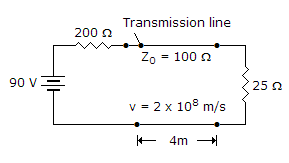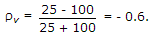# Electronics and Communication Engineering - Microwave Communication

### Exercise :: Microwave Communication - Section 1

11.

In a directional coupler

 A. isolation (dB) equals coupling plus directivity B. coupling (dB) equals isolation plus directivity C. directivity (dB) equals isolation plus coupling D. isolation (dB) equals (coupling) (directivity)

Explanation:

Isolation equals coupling plus directivity.

12.

As the frequency is increased, the charging MVAR in a cable

 A. decreases B. increases C. remain the same D. decreases or remains the scheme

Explanation:

Charging current and MVAR are proportional to frequency.

13.

Loss angle of a good quality cable is about

 A. 1° B. 30° C. 70° D. 90°

Explanation:

For ideal dielectric loss angle is zero. For good quality cables, loss angle is very small, about 1° only.

14.

In a three cavity klystron amplifier, the oscillations are excited in

 A. input cavity B. output cavity C. intermediate cavity D. both (a) and (b)

Explanation:

A Klystron is a vacuum tube used for generation/amplification of microwaves.

An electron beam is produced by oxide coated indirectly heated cathode and is focussed and accelerated by focussing electrode.

This beam is transmitted through a glass tube. The input cavity where the beam enters the glass tube is called buncher.

As electrons move ahead they see an accelerating field for half cycle and retarding field for the other half cycle.

Therefore, some electrons are accelerated and some are retarded. This process is called velocity modulation.

The velocity modulation causes bunching of electrons. This bunching effect converts velocity modulation into density modulation of beam.

The input is fed at buncher cavity and output is taken at catcher cavity.

In a two cavity klystron only buncher and catcher cavity are used. In multi cavity klystron one or more intermediate cavities are also used.

The features of a multicavity klystron are :

1. Frequency range - 0.25 GHz to 100 GHz

2. Power output - 10 kW to several hundred kW

3. Power gain - 60 dB (nominal value)

A multicavity klystron is used in UHF TV transmitters, Radar transmitter and satellite communication.

15.

In the given figure reflection coefficient at load isA. 0.6 B. - 0.6 C. 0.4 D. - 0.4